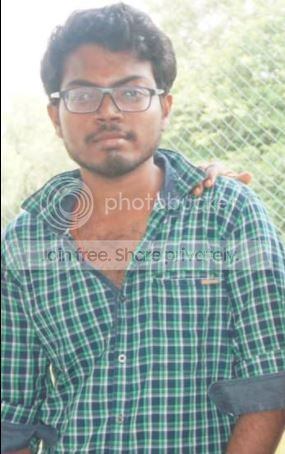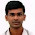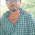# Pharma Engineering

An Engineer's Choice

• ## Wednesday, 17 October 2018

Hiii all......!!!

After a gap back with a case study, First of all sorry for being non-responsive towards the comments that i've been receiving.

Being little busy, i'm unable to concentrate on the blog,

Anyway, i'm adding a Forum page, where you can add your queries and those don't need my approval, and so that anyone who are well aware of the query topic can attend your query.

I've received a query requesting to design a distillation setup which will be of ideal features including line sizes.

Another query that i've received very rapidly these days is about Ejectors, and i'll share about the ejectors in my next post.

So, i'll mention you how to design an ideal setup for reactor distillation by combining some of the posts that i've already shared with you.

The case is that Methanol need to be distilled out from a 5 KL reactor having 80% occupancy, the end point of distillation is retaining 1000 L of reaction mass.
Current Scenario: Existing time-cycle of distillation is 12 hours,
Required Scenario: Expected time-cycle of distillation is 6 hours.

The expected case may seems to be quite typical, but it is possible.

Existing rate of distillation = (4000 - 1000) / 12 = 250 Lts/hr,
Expected rate of distillation = (4000 - 1000)/6 = 500 Lts/hr.
Now we'll start from selecting vacuum pump capacity & line sizings,
Total expected system volume will be 8000 L,  [which includes 4000 L reactor, 1000 L receiver, Condensers holdup, vacuum trap, lines holdup],
So before calculating the vacuum pump capacity, we need to perform vacuum leak test,
Lets suppose we have 6 mmHg of vacuum drop for whole system in a minute.

STEP - 1 :

So, air-leakage rate shall be 800 torr.Lt/Sec.

Lets suppose the required ultimate pressure on the system be 10 torr.
Vacuum pump capacity shall be 288 m3/hr, [Refer Vacuum pump capacity calculation here]

For this we shall be considering 30 % of excess as a service factor.
So the pump capacity shall be 374.4 m3/hr ~375 m3/hr.

Now there should be a line from vacuum pump to the system,
the line size shall be 1.83 inch, Considering excess it shall be ~2.5"/3".

Now the Vacuum pump & line from vacuum pump to condenser is ready,

STEP - 2:

Now we need to calculate the condenser capacity required for condensing the 500 Lts/hr of methanol.
Expected volumetric flowrate of methanol is 500 Lts/hr,
Expected mass flowrate of methanol is 500 x 0.792 = 396 Kgs/hr.

Lets suppose we have chilled brine circulation with an inlet temperature of -20 C,
Let the outlet temperature be -10 C.
Let the vacuum on the system be 680 mmHg,
Vapour temperature at 680 mmHg is 16.39 C

LMTD = 33.83 C, [Refer LMTD calculation here]

Consider the overall heat transfer coefficient be 300 KCal/hr.m2.C,

Primary condenser area shall be 10.30 m2,
Considering service factor 30%, the area shall be 13.39 m2 ~15 m2

Secondary condenser area shall be considered as 40% of primary condenser,
required area shall be 13.39 x 0.4 = 5.36 m2 ~6 m2.

By here, condenser capacity requirement is evaluated.

STEP - 3:

Now, we need to calculate the vapour line sizing,

Total expected boil-up is 500 Lts/hr = 396 Kg/hr = 871.20 lbs/hr.
Methanol boiling point at 680 mmHg is 16.39 °C = 521.18 °R,
Methanol mol. wt. 32.04 g/mole,
Volumetric flow of solvent vapour is 1634.14 ft3/hr.

Air load at the location be 2 Kg/hr, volumtric flow shall be 9.19 ft3/hr.

Total volumetric flowrate be 1634.14 + 9.19 = 1643.32 ft3/hr.

Vapour velocity at 680 mmHg shall be ~170 ft/sec = 51.82 m/sec.
This i got from thumb basics, so i'll be unable to explain you guys, Sorry for that.

Due to the pipe roughness factor, there shall be loss in velocity of vapour, it'll be in range of 20-30%, lets consider 25%,
the vapour velocity will be 51.82 x 0.75 = 38.86 m/sec = 127.5 ft/sec

Vapour column size = 0.52 ft = 6.55" ~7"

Now we need to calculate the line size between primary condenser and secondary condenser,

Usually the secondary condenser is used to condense the uncondensed vapours escaping from the primary, the escaping volume shall be around 20%.

So the total volume that enters the secondary condenser shall be 1643.32 x 0.2 = 328.67 ft3/hr.

Line size required is 0.146 x SQRT(328.67/170) = 0.23 ft = 2.93" ~3"

Now, we need to calculate the line required for transferring of the condensate from condenser to collection receiver.

the expected collection is 500 Lts/hr,
Let the velocity of the fluid under gravity be 1.5 m/sec.

Line size required is 1.3" ~1.5"/2"
This is a damn basic calculation, formula used is Q = velocity x Cross sectional area.

by now, line sizes within the distillation system were done.
STEP - 4:

Hot water system requirement & Plate heat exchanger capacity requirement for hot water system.

Total heat energy required for achieving the boil-up = 106128 Kcal/hr.
Formula used is a general thing, (M x Cp x dT) + (M x ƛ).

Lets suppose the difference between jacket in & out temperatures be 3 C,
Specific heat of water, Cp = 1 KCal/Kg.C,
Velocity of utility in jacket be 3 m/sec,
Total flowrate required is 106128/(1 x 3 ) = 35376 Kgs/hr = 35.376 m3/hr ~40 m3/hr
Pump capacity is 40 m3/hr.

Motor capacity required is 1108.6 watts = 1.49 HP
Considering the service factor 0f 30%, capacity shall be ~2.5 HP/5 HP

Steam in temperature is 120.5 @1Kg/cm2

Overall heat transfer coefficient of PHE is 400 KCal/hr.m2.C.

Let the hot water set point be 50 C,
PHE capacity is 3.52 m2 ~4 m2
[Formula used for the calculation is Q = U x A x LMTD]

That's it........!!! The distillation system is ready, and it can meet the requirement of enhancing the boil-up & can efficiently reduce the time-cycles,

I cant guarantee the calculated system can reduce the time cycle to 6 hours, but it will be effective.

Any queries feel free to comment / message.

Comments are most appreciated.Reference ScreenShot

Related Posts:
[How to] Select a filter for pharma operation ?
[How to] Calculate vapour column height required ?
[How to] Calculate heat of reaction from bond energy calculations ?
[How to] Map equipment's for a manufacturing process ?Hi! I am Ajay Kumar Kalva, Currently serving as the CEO of this site, a tech geek by passion, and a chemical process engineer by profession, i'm interested in writing articles regarding technology, hacking and pharma technology.
Follow Me on Twitter AjaySpectator & Computer Innovations

1.Hai Ajay, Can u plz calculate the setting time during extraction/ work up with case study ( example) .

Regards
Hari
harikrishnap018@gmail.com

1.Dear HariKrishna,

Regards,
AJAY K

2.Dear Ajay,
i am so glad to have a blog like this. I am learning a lot from ur writings
i have a doubt regarding the pump capacity calculation
S.avg = 288 m3/hr
and am getting 288 Lt/Sec = 288*3600/1000 = 1036.8m3/hr . Does my conversion has any mistake

Regards,
A.Dorababu

1.Dear Dorababu,

Air leakage rate i've taken is 800 Torr.Lt/Sec,
From this, i'll be calculating the pump capacity with an ultimate pressure of 10 torr vacuum, so it will be 800/10 = 80 Lt/Sec = 80 x 3600 / 1000 = 288 m3/hr.

From the above comment, i can tell that you have considered the pump capacity in air-leakage rate. That's the error.

Best Regards,
AJAY K

3.ultimate pressure of 10 torr vacuum means , is it vacuume required to distilled solvent or something else?

1.Ultimate means its the final/maximum vacuum that can be obtained using a pump.

4.Can Improper cooling to the condenser reduce the distillation rate under vacuum distillation or will it be the same??

1.Of course, Inadequate cooling might lead to accumulation of un-condensed vapours, which might result in vacuum drop. Whereas a right flow of utility does the job in obtaining optimum distillation rates.

** Next time kindly comment with you good name please

Best Regards,
AJAY K

5.Thank you Very much Mr Ajay
Regards
AkshayHi! I am Ajay Kumar Kalva, Currently serving as the CEO of this site, a tech geek by passion, and a chemical process engineer by profession, i'm interested in writing articles regarding technology, hacking and pharma technology.